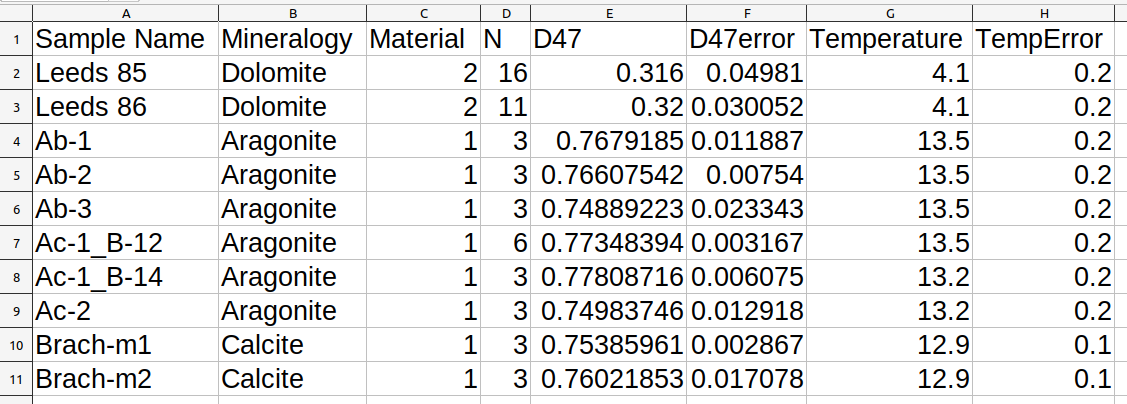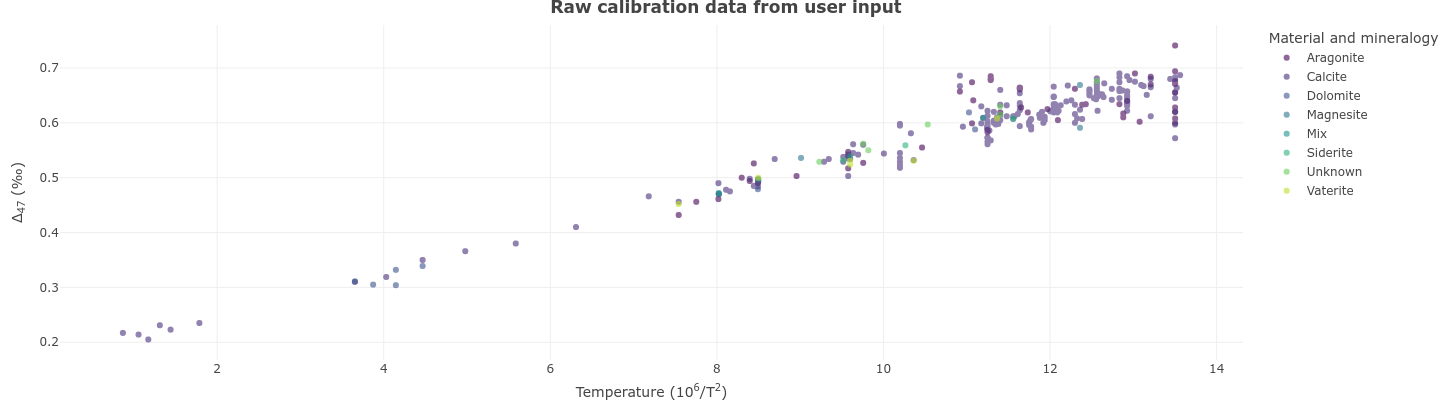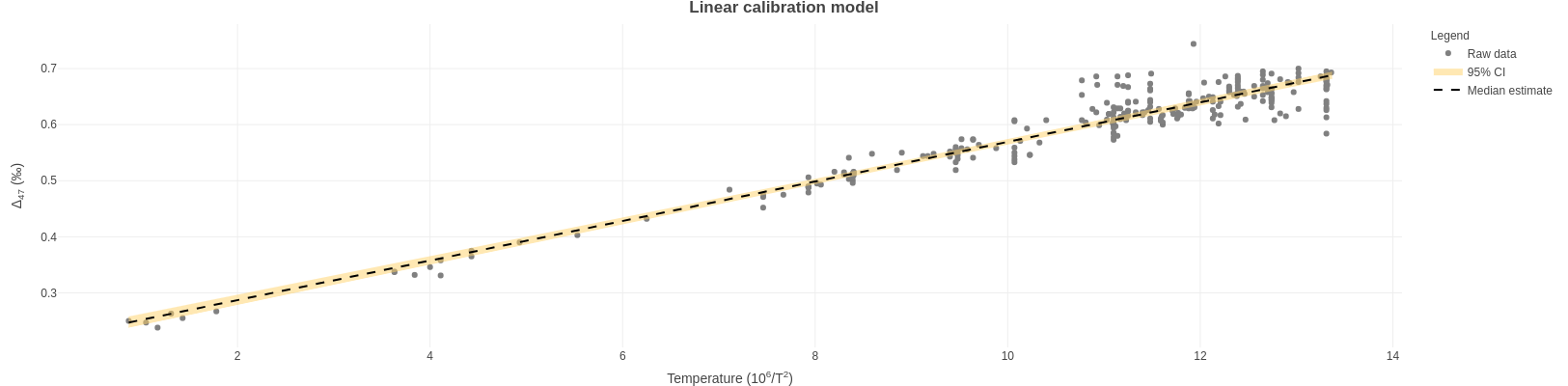# Welcome to BayClump!

### Bayesian methods for clumped isotope paleothermometry# Calibrations

#### Step 2: Select Models

#### Linear model

#### Inverse weighted linear model

#### York regression

#### Deming regression

#### Bayesian simple linear model - no errors

#### Bayesian simple linear model - with errors

#### Bayesian mixed model - no errors

# Reconstructions tab

#### Step 1: Reconstruction setup

Models to run:

# BayClump Basics

BayClump version 0.9.2 was developed in R version 4.1.2 and RStudio version 1.4.1106. It has been tested up to R 4.2.1 and RStudio 2022.02.3+492. This app accompanies Román-Palacios et al. under revision: BayClump: Bayesian Calibration and Temperature Reconstructions for Clumped Isotope Thermometry. Link.

BayClump is separated into calibration and reconstruction workflows. Any calibration models selected on the Calibrations tab are automatically made available for use in the Reconstructions tab. At this time, only $$Δ_{47}$$-based calibrations and reconstructions are supported.

Please review the documentation presented under the user manual before using the app. We note that the code provided in the app is also available in an R package, bayclumpr.

# Calibration data

Two calibration datasets are included in BayClump by default. Dataset 1 is based on the reprocessed datasets of Petersen et al. (2019). Dataset 2 is based on the reprocessed and original datasets of Anderson et al. (2021). The datasets from Petersen et al. (2019) have been reprojected into the I-CDES reference frame after Bernasconi et al. (2021) at a growth temperature of 90°C, so as to be compatible with the datasets of Anderson et al. (2021). Throughout, we refer to this as $$I-CDES_{90}$$.

The default calibration datasets may be used individually or in combination to create calibration models, and may also be combined with the user's own calibration data in the I-CDES 90 reference frame. The user may also choose to work exclusively with their own calibration data in $$I-CDES_{90}$$ or another reference frame if desired. At this time, BayClump does not provide the option to reproject the default calibration datasets into a different reference frame.

BayClump provides a template for users to upload calibration data. It can be downloaded from the Calibrations tab. Sample data are shown in the first ten rows of the template and should be overwritten. A few important notes on the structure of your calibration dataset:

• The template must be left in .csv format, although the user is free to change the name of the spreadsheet as needed. Do not change column headings or orders.
• Do not enter extra columns - these will not be used by the app and may create problems.
• Please make sure that uncertainties are all > 0. The app will not run otherwise and will print a message to the console.
• The dataset MUST present sample-level observations. Rows are not expected to reflect replicates per sample.
• If a sample was analyzed under a single replicate, please assign the instrument's uncertainty as the error.
• BayClump assumes that uncertainties in the calibration dataset for temperature and $$Δ_{47}$$ are in standard deviation.Below, we provide a brief description of the columns that are required in the calibration dataset.

• Sample Name: This should be a unique identifier for each sample. Combinations of letters, numbers, spaces, and special characters may be used. It is best to avoid number signs (#) as this may cause the code behind BayClump to malfunction.

• Mineralogy: This should be the full, capitalized name, i.e., Aragonite, Calcite, Dolomite, Mixed, Unknown, etc.

• Material: This must be entered as a number. If you are using any of the preloaded calibration sets, be sure to follow the convention 1 = Natural, 2 = Synthetic, and 3 = Biogenic to match those datasets. Additional numbers may be used as necessary. If you are using strictly your own calibration data, you can select your own convention. We only ask that the sequence of materials that you select must start at 1 and that you should not skip numbers (e.g. Incorrect: 1 = Natural, 3 = Synthetic).

• N: The number of replicates measured to produce the final value. This must be numeric - no letters or special characters are accepted.

• D47: The final $$Δ_{47}$$ value of the sample after completing all appropriate quality assurance steps. This must be numeric - no letters or special characters except for periods and minus signs are accepted. Provide as many decimal places as possible to improve calculations.

• D47error: The standard deviation around $$Δ_{47}$$ after completing all appropriate quality assurance steps. This should be the combined measurement and correction error. This must be numeric - no letters or special characters except for periods are accepted. Provide as many decimal places as possible to improve calculations. Please make sure that this column has no negative numbers.

• Temperature: Growth temperature in $$10^6/T^2$$, where temperature, T, is in Kelvin. This must be numeric - no letters or special characters except for periods and minus signs are accepted.

• TempError: Standard deviation around the growth temperature in $$10^6/T^2$$, where temperature, T, is in Kelvin. This must be numeric - no letters or special characters except for periods are accepted. Please make sure that this column has no negative numbers.

## Calibration Model Setup and Selection

BayClump offers five calibration model options. Bootstrapping is used to produce robust estimates of regression coefficients for non-Bayesian models. The user is able to type in the number of replicates or use the default 100 bootstrap. We recommend that the number of bootstrap replicates chosen be at least 10 times the number of unique samples, with a minimum of 50 and a maximum of 1,000. For example, if you have 10 unique samples you wish to use in calibration models, you should choose at least 100 bootstrap replicates (10 x 10). If you have 3 or fewer samples, do not exceed 50 bootstrap replicates.

• Warning: Selecting all available calibration models and a large number of bootstraps will require significant processing time. This may exceed 30-40 minutes if server traffic is heavy. The app may appear to hang while calculations are being performed, but should complete normally. Do not disconnect from the app during the calibration run

• Important: Calibration data should be entered into the template in $$10^6/T^2$$ format, where temperature, T, is in Kelvin.

### Number of posterior samples for Bayesian models

The number of iterations needed to allow models to converge properly varies by dataset. The number of retained posterior samples will likewise vary, but the default of 3,000 will be appropriate for most applications. Reduce this number to improve times, or increase the number if the models are having issues converging. Increasing the number of posterior samples takes more time.

### Number of observations per bootstrap sample

This parameter allow users select the number of replicates to be included in each bootstrap replicate. For instance, users can choose to select the total number of samples to be analyzed per bootstrap (sampled with replacement). Users can also choose to fit each bootstrap based on a limited number of samples of the calibration dataset.

## September 26th, 2022 – *BayClump v 1.0.0

BayClump now runs on stan!

We have fully migrated BayClump's Bayes engine from JAGS 4.3.0 to stan 2.30.0 (https://mc-stan.org/) via the rstan package (http://mc-stan.org/rstan/). This change speeds up calculations and improves overall performance. Furthermore, we have standarized all the user inputs and BayClump's outputs to increase the consistency of the app. Users are expected to provide uncertainty in the form of standard deviations. BayClump will provide results on parameter uncertainty in standard error and temperature reconstruction in standard deviation. Below, we provide a list of additional changes in different tabs.

Changes to the Calibration tab:

• You can now select the number of Bayesian iterations to keep rather than setting a total number of Bayesian iteration to run (default is 3,000)
• You can now select to use Informative, Weakly Informative, or Uninformative priors for fitting Bayesian models. We recommend using Weak priors.
• Model selection for Bayesian models now uses log likelihoods from loo 2.5.1 (https://mc-stan.org/loo/)

Changes to the Reconstruction tab:

• Removed option for the Classic Calibration Approach
• Uncertainty is presented in 1 SE

## March 28th, 2022 – BayClump v 0.9.1

We have a News and Updates section now! Stay tuned for info on what's new with BayClump. Updates to functions

The BayClump team is grateful to Ilja Kocken, author of the clumpedr package, for alerting us to a problem with the way clumpedr's revcal function propagates uncertainties and deals with covariance between slope and intercept. You can see the issue on BayClump's GitHub repo here: Issue #7 and the subsequent update to clumpedr here on Ilja's GitHub repo.

The latest version of BayClump propagates uncertainties using two alternative approaches that are outlined in detail in our paper. In general, the inversion process for estimating $$T_{(^\circ C)}$$ follows the common practice in the field:

$T_{(^\circ C)}= \sqrt{\frac{\beta * 10^6}{\Delta_{47} - \alpha}} - 273.15$

Temperature error is propagated in a Bayesian framework based on parameter uncertainty and uncertainty in the analyzed $$\Delta_{47}$$ using the posterior distribution of parameters during the calibration step and a prior distribution on temperature. For non-Bayesian models, uncertainty is calculated as the difference between the point estimates of $$T_{(^\circ C)}$$ for $$\Delta_{47}$$ and $$\Delta_{47} + SE(\Delta_{47})$$.

## Pre March 28th, 2021 – BayClump v 0.9

Lots has changed with this update!

Additions to the Calibration tab include:

• A slider that allows users to select the temperature range for estimation of confidence intervals
• The option to change the number of iterations to keep for Bayesian models (default is 3,000)
• The option to multicore Deming regressions to improve computing time
• A menu to select either informative or diffuse priors for Bayesian models
• More detailed progress bar messages

Additions to the Reconstruction tab include:

# The Reconstructions Tab

BayClump provides a template for users to upload reconstruction data. It can be downloaded from the Reconstructions tab. Sample data are shown in the first ten rows of the template and should be overwritten. A few notes on the structure of the reconstruction file:

• The template must be left in .csv format, although the user is free to change the name of the spreadsheet as needed.
• Materials (i.e. numbers) must correspond to those used in the Calibrations tab. Users can skip numbers in the Reconstructions tab (not allowed in the Calibrations tab).
• Do not change column headings or orders. Do not enter extra columns - these will not be used by the app and may create problems.
• The dataset MUST present sample-level observations. Rows are not expected to reflect replicates per sample.
• If a sample was analyzed using a single replicate, please assign the instrument's uncertainty as the error.
• BayClump assumes that uncertainties in that target $$\Delta_{47}$$ are in standard deviation

# The Calibration Plots Tab

All plots are created in plotly version 4.9.3 (Sievert 2020). They are fully interactive and downloadable. Plots download at web viewing resolution (72 dpi), 1244x400 pixels, and in .png format by default, but may be scaled and resized in external software if desired.The Calibration Plots tab will display raw calibration data based on user input. The default display is of calibration data for Model 1. It will automatically update to reflect the choice of calibration data made on the Calibrations tab.

Once calibration model runs are complete, the Calibration Plots tab will update to show plots of raw data on the $$10^6/T^2$$ temperature scale, with overlaid 95% confidence intervals (credible intervals in the case of the Bayesian model), and a median line.# Calibration model descriptions

Note: The descriptions provided below are taken from the publication this app accompanies. For additional information, please see Román-Palacios et al. under revision.

## Simple linear model

This regression model is the simplest model used in this study. The simple linear regression does not explicitly account for measurement error in $$10^6/T^2$$. We fit the simple linear regression model using the lm function in the stats R package under default parameters. Note that the approach implemented in the lm function in R is based on least squares. Under least squares, the resulting regression model is selected as the line that minimizes the sum of squared error (i.e., sum over the squared differences between the points and the line) in the relationship between $$10^6/T^2$$ or $$Δ_{47}$$.

## Inverse weighted linear model

Simple linear regression fit under least squares, with observations being weighted based on the inverse of their squared uncertainty in the residual $$Δ_{47}$$. Note that, although this approach indirectly accounts for uncertainty in $$Δ_{47}$$, weighted models analyzed in this study do not explicitly account for measurement error in $$10^6/T^2$$. The weighted simple linear regression was fit using the lm function in the stats R package.

## Deming regression

The Deming model also fits under least squares, and the optimization steps while fitting the straight line follow the algorithm of Deming (1943). This model explicitly accounts for measurement error in both $$Δ_{47}$$ and $$10^6/T^2$$. Under the Deming regression, the ratio of the weights (related to their uncertainty) in $$Δ_{47}$$ and $$10^6/T^2$$ is constant over all data points (Martin 2000). To fit this model, we specified observations in $$Δ_{47}$$ and $$10^6/T^2$$, along with the corresponding error in each observation. Note that although the Deming model also fits under least squares, the optimization steps while fitting the straight line follows the algorithm presented in Deming (1943).

## York regression

The York model is closely related to the Deming regression model. However, under the York model, the ratio of the weights (related to their uncertainty) in $$Δ_{47}$$ and $$10^6/T^2$$ varies over data points (Martin 2000). Therefore, the York model is slightly more complex than the Deming regression model. We specified observations in $$Δ_{47}$$ and $$10^6/T^2$$, along with the corresponding error in each observation, when fitting this regression model.

## Bayesian simple linear model

This model is the simplest Bayesian model currently implemented in the app. This Bayesian model is equivalent to the simple linear regression model. However, instead of parameter estimates based on least squares, optimization of regression parameters is conducted under a Bayesian framework. This model is fit using stan with priors on the slope and intercept defined in the previous section.

## Bayesian linear regression model with error in variables

This model explicitly accounts for measurement error in both $$10^6/T^2$$ and $$Δ_{47}$$ during parameter estimation. The Bayesian linear model with error in variables is close to the classical calibration model used in the field, a York model. This model is fit using stan with priors on the slope and intercept defined in the previous section.

## Bayesian linear mixed model

In some situations, it is desirable to account for potential differences in the relationship between $$Δ_{47}$$ and $$10^6/T^2$$ between a factor (e.g. material, species). Under the Bayesian linear mixed model, parameter estimates are performed in accordance with a specific factor. Therefore, this model could be used to examine for potential differences in slope/intercept for a particular grouping variable of interest. We also note that parameter estimates under the Bayesian linear mixed model account for error in both $$Δ_{47}$$ and $$10^6/T^2$$. This model is fit using stan with priors on the slope and intercept defined in the previous section.

### Bayesian priors

We have implemented three alternative sets of distributions that are used to define the prior distributions on the slope ($$\beta$$) and intercept ($$\alpha$$). Two of the priors include information that was based on a review of parameter estimates from previous calibration studies (see the preprint). The Informative prior reflects the mean and standard deviation in slope ($$\beta$$) and intercept ($$\alpha$$) across the analyzed studies.

$\alpha \propto N(0.231, 0.065)$

$\beta \propto N(0.039,0.004)$

The Weak prior reflects the mean slope ($$\beta$$) and intercept ($$\alpha$$) but has an standard deviation of 1.

$\alpha \propto N(0.231, 1)$

$\beta \propto N(0.039, 1)$ We recommend using Weak priors.